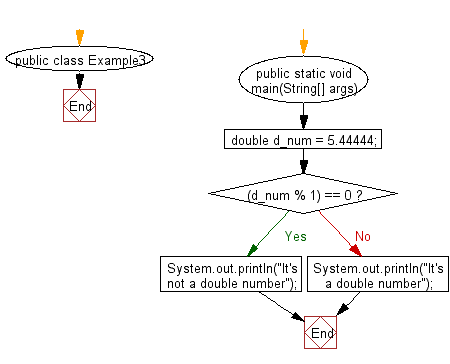﻿ Java Math Exercises: Test if a double number is an integer - w3resource# Java Math Exercises: Test if a double number is an integer

## Java Math Exercises: Exercise-3 with Solution

Write a Java program to test if a double number is an integer.

Sample Solution:

Java Code:

``````import java.util.*;
public class Example3 {
public static void main(String[] args) {
double d_num = 5.44444;
if ((d_num % 1) == 0) {
System.out.println("It's not a double number");
} else {
System.out.println("It's a double number");
}
}
}
``````

Sample Output:

```It's a double number
```

Flowchart:Java Code Editor:

What is the difficulty level of this exercise?

﻿

## Java: Tips of the Day

Parsing dates:

```import java.io.*;
import java.util.*;
import java.text.*;

String s = "2001/09/23 14:39";

SimpleDateFormat formatter = new SimpleDateFormat ("yyyy/MM/dd H:mm");
Date d = formatter.parse(s, new ParsePosition(0));
```